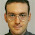## Thursday, November 18, 2010

### How to do better on physics exams

Since I am marking many first year exams here are a few tips. They are all basic but it is amazing and discouraging how few students do the following:

Clearly state any assumptions you make. Don't just write down equations. e.g, explicitly state, "Because of the Newtons second law ..." or "we neglect transfer of heat to the environment."

Keep track of units at each stage of the calculation. Don't just add them in at the end. All physical quantities DO have units. If you make a mistake it will often show up in getting the wrong units.

Clearly state the answer you obtain. Maybe draw a box around it. e.g, "The change in entropy of the gas is 9.3 J/K".

Try and be neat and set out your work clearly. If you make a mess just cross out the whole section and rewrite it. I see many students exam papers where I really have no idea what the student is doing. It is just a random collection of scribbles, equations, and numbers....

Think about the answers you get and whether they make physical sense. e.g. if you calculate the efficiency of the turbine is 150 per cent or that the car is travelling at 1600 metres per second you have probably made a mistake!

1.I'm actually a bit skeptical of the claim that "All physical quantities DO have units." If I say that a quantity increases logarithmically with distance, say log(kd), then that's log(k)+log(d). But what does it mean to take the log of a metre? I think that maybe the quantities have no units...

2.this is the reason why you put the k, which will have units of m^-1, so that the argument of logs exps and trigonometric functions are appropriately dimensionless.

3.But log(kd)=log(k)+log(d), so the log is applying to the metres directly. Also, e^(kd)=(e^d)^k. In maths we always make quantities dimensionless...

4.Hmmm. The log of a product is the sum of the logs if the arguments are numbers. What if they are not? Presumably d is the norm of a vector which can be written d^T.d. What if kd was written as d^T.k.d? where d is a vector, d^T its transpose, and k a matrix? Are you automatically justified in making the log of the product a sum of the logs?

5.I think it would have to be something more like sqrt(d^T.k^2.d). But if we're not allowed to write log(kd)=log(k)+log(d), then that would needlessly complicate many things. Maybe log(m) and e^m are units themselves, just as m^2 & m^3 are. I have no idea what they'd represent physically, though...